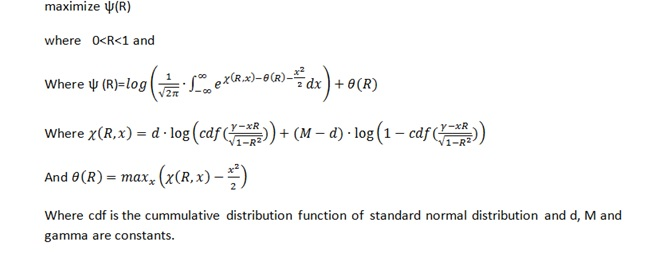## maximization in proc iml with global variable

hi all,

i would like maximize a function that is similar to this: -(x-2*r)**2/2+3*r  for x with global variable r in proc iml and to be able to use the result in a further step in iml.

(the expected result would be 2r)

i started the code:

proc iml;

start f(x) global(r);

f=-(x-2*r)**2/2+3*r;

return(f);

finish f;

x0j={0.};

optnj={1 3};

call nlpcg(rc,xres,"f",x0j,optnj);

quit;

but i got the following error message:

98   proc iml;

99   start f(x) global(r);

100      f=-(x-2*r)**2/2+3*r;

101      return(f);

102  finish f;

NOTE: Module F defined.

103  x0j={0.};

104  optnj={1 3};

105  call nlpcg(rc,xres,"f",x0j,optnj);

ERROR: (execution) Matrix has not been set to a value.

is there a way to do the optimization and save the result as a module? Thanks for any help!

Anita

12 REPLIES 12

## Re: maximization in proc iml with global variable

You did not define r. The objective function uses r, so if it is not defined it will give you an error.

## Re: maximization in proc iml with global variable

Thanks for the answer! But my problem is that i cannot define r with a closed formula. I only know abt it that it should be between 0 and 1.  I will use the result of this maximization in a further step to define another function and will optimize that function for r, so at this step i cannot define r. Is there a way to have a result without specifying r? so the result would not be a value but a formula depending on r.

Thanks!

## Re: maximization in proc iml with global variable

Take the derivative of the expression. Set it equal to zero and solve for x.  That gives the optimal value as a formula that depends on r.

## Re: maximization in proc iml with global variable

That would be great! Is there a way to calculate the derivatie in sas iml? I tried it with nlpfdd but i have the same problem as above.. Thanks for the help!

## Re: maximization in proc iml with global variable

Could you give us or point us to a complete mathematical description of your problem?

## Re: maximization in proc iml with global variable

SAS/IML is software for numerical computations; it does not perform symbolic algebra. You can use a program such as Mathematica or Maple for symbolic computations.  You might like to read the article Optimizing? Two hints for specifying derivatives - The DO Loop, which also discusses using the free Wolfram Alpha software to compute symbolic derivatives.

In theory, derivatives are easy to compute, provided that the function is not too complicated and has an analytical expression.

Inverting the expression df/dx=0 is usually the hard part, since it is a root-finding problem. The NLP functions enable you to solve the problem without computing the derivatives or solving for roots.

I agree with Joe: say more about what you are trying to accomplish.  For example, are you trying to optimize the function for various values of r?  That is easy to accomplish by looping over the range of r.

## Re: maximization in proc iml with global variable

thank you for your help! i will try the do loop for the calculation. i was just wondering if there is a different way. You can find my problem below:thanks!

## Re: maximization in proc iml with global variable

Wow. That's a heck of a problem!  Here are some observations:

1) This is an optimization problem in R. The x variable gets integrated out. So the objective function is Psi(R).  That is the function to call from the NLP routines.

2) For each call to Psi, R is known. It equals some value, so from within Psi you can find Theta. That might involve a separate computation that maximizes Chi(x) - x##2 / 2.  I've never tried to optimize a second function while I'm in the middle of optimizing the first function, but if necessary you can precompute Theta(R) on a grid of R values.

3) When you are computing Chi, use the LOGCDF function to compute the first term and the LOGSDF function to compute the second term.

4) I do not know an easy way to integrate the integral inside the LOG function.  Does it have a name or do you know any more information about it?  To me, that looks like the hard part of this problem.

I have written two blog posts that discuss optimizing a function that evaluates an integral:

## Re: maximization in proc iml with global variable

Do you have values for m, D, and gamma?

## Re: maximization in proc iml with global variable

here are an example set of values for m, d and gamma

M:30329

gamma: -2.337336246

d: 252.48226708

thanks!

## Re: maximization in proc iml with global variable

thanks for the tip with the do loop, i could calculate maximum (i have to have a rather precise value as a result so i calculated it for 200 rs and selected the maximum based on that), but i have 300 sets of d,M and gamma, so if i calculate for all of them with the do loop it takes a lot of time and that is why it would be great to be able to do it otherwise.

## Re: maximization in proc iml with global variable

i calculated the integral with quad function and then used the log function for the result of the quad like this:

proc nlp out=solve_x;

max chi_comma;

decvar x;

p_hat=CDF('NORMAL',(&gamma.-x*&r.)/((1-(&r.**2))**0.5),0,1);

chi=&d.*log(p_hat)+(&m.-&d.)*log(1-p_hat);

chi_comma=chi-(x**2)/2;

run;

data _null_;

set solve_x;

call symput("theta", chi_comma);

run;

%put max of chi_comma is &theta;

proc iml;

pi = constant("pi");

start toint(x);

p_hat=CDF('NORMAL',(&gamma.-x*&r.)/((1-(&r.**2))**0.5),0,1);

p=exp(&d.*log(p_hat)+(&m.-&d.)*log(1-p_hat)-&theta.-x**2/2);

return(p);

finish;

interval = .M || .P;

print pm[format=E21.14];

psi=(log((1/((2*pi)**0.5))*pm)+&theta.);

print psi[format=E21.14];

quit;

From The DO Loop